# Interpolation Formulas

The following article is from The Great Soviet Encyclopedia (1979). It might be outdated or ideologically biased.

## Interpolation Formulas

formulas that give an approximate expression for the function y = f(x) with the help of interpolation, that is, through an interpolation polynomial Pn(x) of degree n, whose values at the given points x0, x1 …, xn coincide with the values y0, y1, …, yn of the function f at these points. The polynomial Pn(x) is uniquely determined, but depending on the problem it is convenient to write it in different forms.

(1) Lagrange’s interpolation formula: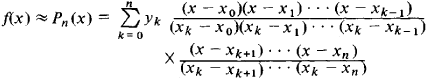The error introduced by replacing the function f(x) by the expression Pn(x) does not exceed in absolute magnitude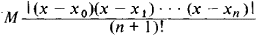where M is the maximum of the absolute magnitude of the (n + 1) th derivative fn + 1 (x) of the function f(x) on the interval [x0, xn].

(2) Newton’s interpolation formula: If the points x0, x1, …, xn are situated at equal distances from each other (xk = x0 + kh), the polynomial Pn(x) may be written as follows: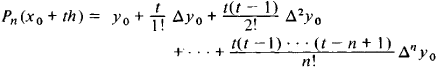(here x0 + th = x, and Δk is the kth order difference: Δkyi = Δk − 1yi + l − Δk − 1yi). This is Newton’s formula for forward interpolation; the name of the formula indicates that it contains given values of y corresponding to the nodes of interpolation located only to the right of xo. This formula is convenient for the interpolation of functions for values of x close to x0. For interpolation of functions for values of x close to xn, a similar Newton’s formula is used for backward interpolation. For the interpolation of functions for values of x close to xk, Newton’s formula is best transformed by an appropriate change of the indexing (see Stirling’s and Bessel’s formulas below).

Newton’s formula can also be written down for unequally spaced nodes by resorting to divided differences. In contrast to Lagrange’s formula, where each term depends on all the nodes of interpolation, any kth term of Newton’s formula depends on the preceding nodes (from the beginning of the indexing), and the addition of new nodes causes only the addition of new terms to the formula (in this lies the advantage of Newton’s formula).

(3) Stirling’s interpolation formula: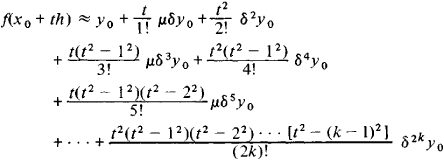Stirling’s formula is used for the interpolation of functions for values of x close to one of the middle nodes a; in this case it is natural to take an odd number of nodes x. k, …, x _1, x0, x1, …, xk, considering a as the central node x0.

(4) Bessel’s interpolation formula: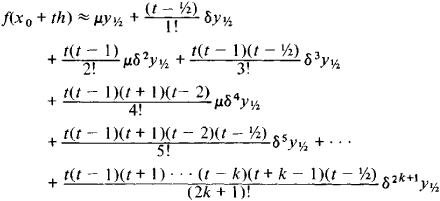is used for the interpolation of functions for values of x close to a middle value a between two nodes; here it is natural to take an even number of nodes xk, …, x−1, x0, x1, …, xk + 1 and to distribute them symmetrically with respect to a(x0 < a < xi).

B. I. BITIUTSKOV

References in periodicals archive ?
In [21, Section 1.5], Phillips has studied two new interpolation formulas on q-integers.
MASJED-JAMEI, On constructing new interpolation formulas using linear operators and an operator type of quadrature rules, J.
Obviously, the interpolation formulas coefficients must be independent from F ([omega]), which is the quantity to find [2, 5].
These formulas are independent of the true value of the spectrum, and then the interpolation formulas are obtained by Fourier series developments.
"Appendix A: Interpolation Formulas for the Adjustment of Index Numbers.
The subject of the talks were in the areas of graph theory, mathematical interpolation formulas, estimating data errors in geographic information systems, how to rank computer performance, how to compare of databases of genetic information, how to write programs to make databases from computer surveys, and image feature recognition in remote sensing.
Computational procedures for the two types of data are generally different because interpolation formulas are often necessary when dealing with frequency distributions.
As an application of the previous results, in Section 3, we obtain Hermite interpolation formulas for nodal systems on [-1,1].
 --, On constructing new interpolation formulas using linear operators and an operator type of quadrature rules, J.
In this section, we construct nonpolynomial (but polynomial based) interpolation formulas at Xu-like points on bivariate domains with different geometric structures, by means of suitable transformations.

Site: Follow: Share:
Open / Close